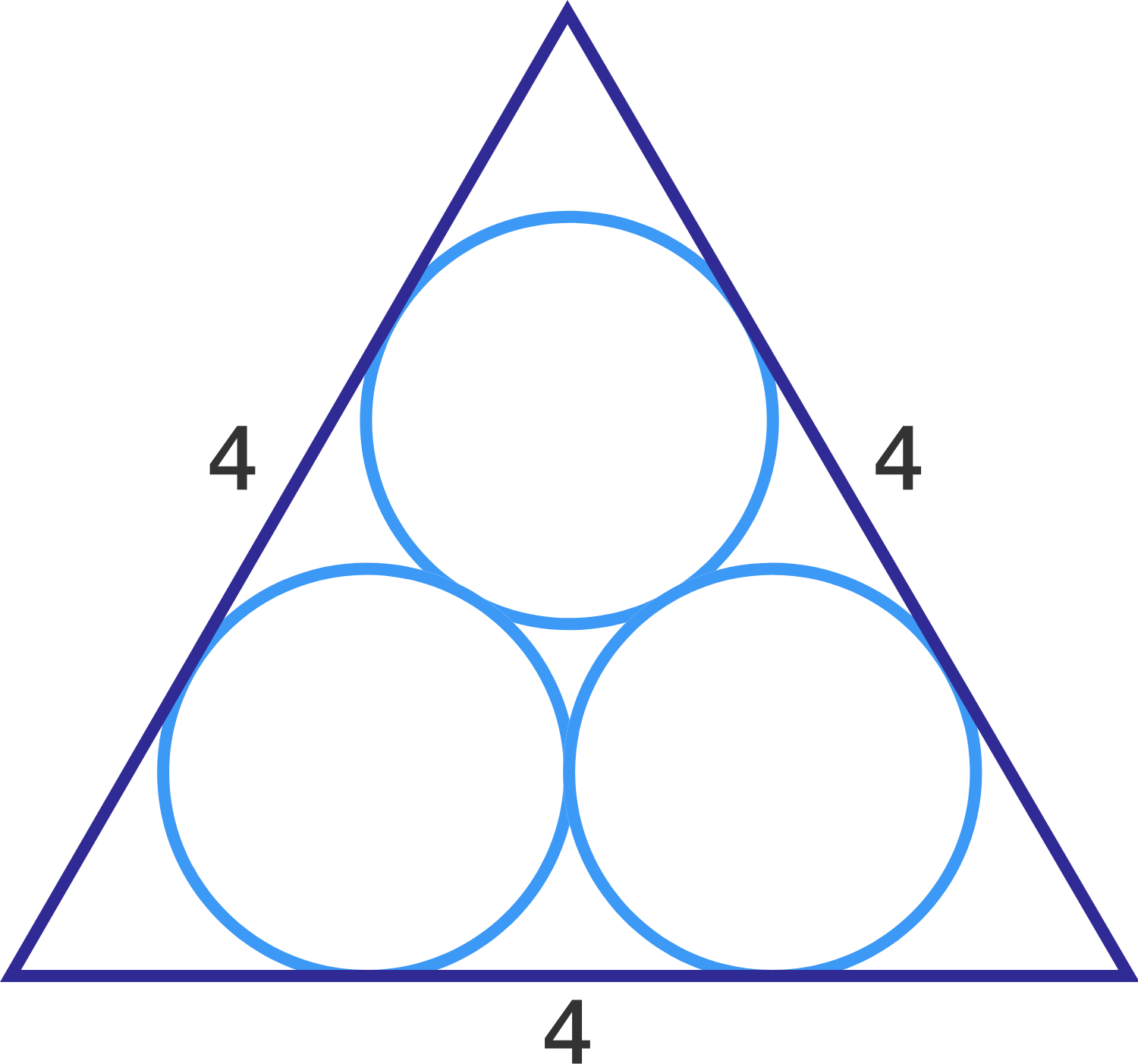Circles in a Triangle 01

Geometry Level 3In the figure, each of the three circles is tangent to the other two and each side of the equilateral triangle is tangent to two of the circles.

If the length of one side of the triangle is 4, what is the radius of each circle?

Details and assumptions:

$\bullet$ All the three circles have equal radii.

×